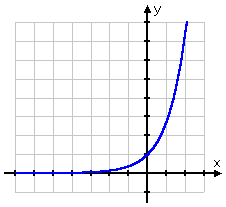Page 35 of 37

### Re: exp(5) = [$]e^5[$]

Posted: April 22nd, 2018, 12:23 pm
What I'm really saying I suppose: I did not come down in the last shower.

### Re: exp(5) = [$]e^5[$]

Posted: April 22nd, 2018, 7:42 pm
I think that you won't get a response unless you challenge them publicly.

What I'm really saying I suppose: I did not come down in the last shower.
I hope that's not an answer you'd give in a live seminar### Re: exp(5) = [$]e^5[$]

Posted: April 23rd, 2018, 7:54 am
What I'm really saying I suppose: I did not come down in the last shower.
I hope that's not an answer you'd give in a live seminarNever had a reason to. In speakers, honesty is the best policy. Don't dig yourself into a hole.

### Re: exp(5) = [$]e^5[$]

Posted: April 23rd, 2018, 10:31 pm
Sure. What does it have to do with the question whether your answer was relevant? The paper was discussing properties of minimas, not optimisation.

### Re: exp(5) = [$]e^5[$]

Posted: April 24th, 2018, 9:33 am
The paper was discussing properties of minimas, not optimisation.
Not at all; it's about DL-PDE(See the DL-PDE thread). Besides, what is your Venn diagram for the above?

I fear my questions will remain unanswered..

### Re: exp(5) = [$]e^5[$]

Posted: June 19th, 2018, 11:52 am
Prove that [$]e[$] is the number that maximises [$]\sqrt[x] {x}[$] for [$]x > 0[$].

### Re: exp(5) = [$]e^5[$]

Posted: June 19th, 2018, 1:19 pm
Prove that [$]e[$] is the number that maximises [$]\sqrt[x] {x}[$] for [$]x > 0[$].
[$]x^ {1/x}=\exp\left[\frac{\log x}{x}\right][$] so ,maximize [$]\frac{\log x}{x}[$]

### Re: exp(5) = [$]e^5[$]

Posted: June 20th, 2018, 6:32 pm
Prove that [$]e[$] is the number that maximises [$]\sqrt[x] {x}[$] for [$]x > 0[$].
[$]x^ {1/x}=\exp\left[\frac{\log x}{x}\right][$] so ,maximize [$]\frac{\log x}{x}[$]
And if we continue with [$]log x/x < 1 [$] we conclude [$]\sqrt[x] {x} < e [$] for any [$]x>=0[$].

Compare with Steiner's proof in Crelle's journal (overkill?)

http://www2.washjeff.edu/users/mwolterm ... rie/89.pdf

BTW [$]\pi(x) = x/log x[$] is the (conjectured) number of primes that do not exceed x. Collector, are you there?

// The Gozilla number cruncher minimises  [$]- \sqrt[x] {x}[$] as 2.7182818..

### Re: exp(5) = [$]e^5[$]

Posted: June 20th, 2018, 7:12 pm
Compare with Steiner's proof in Crelle's journal (overkill?)

http://www2.washjeff.edu/users/mwolterm ... rie/89.pdf
he does seem to have made a mountain out of a molehill.

### Re: exp(5) = [$]e^5[$]

Posted: July 13th, 2018, 1:15 pm
The only solution left is tie solve this problem using (a hand-crafted?) ML algorithm?

Can I just look at the diagram and give the answer?[$]e^x[$] is difficult with standard GD (bad answers) but [$]e^{-x}[$] is better. But we see how the learning rate is important.
(using sigmoid here)### Re: exp(5) = [$]e^5[$]

Posted: July 13th, 2018, 1:19 pm
cntd### Re: exp(5) = [$]e^5[$]

Posted: September 16th, 2018, 7:43 pm
π⁴ + π⁵ ≈ e⁶
to seven significant figures

Ref
Analysis Fact  @AnalysisFact

### Re: exp(5) = [$]e^5[$]

Posted: September 16th, 2018, 7:56 pm
π⁴ + π⁵ ≈ e⁶
to seven significant figures

Ref
Analysis Fact  @AnalysisFact
"Das ist nicht nur nicht richtig; es ist nicht einmal falsch!".

### Re: exp(5) = [$]e^5[$]

Posted: September 16th, 2018, 9:19 pm
π⁴ + π⁵ ≈ e⁶
to seven significant figures

Ref
Analysis Fact  @AnalysisFact
"Das ist nicht nur nicht richtig; es ist nicht einmal falsch!".
it's true but not necessarily useful. You want [$]e^{5}[$] and this is a formula for [$]e^{6}[$]
Computing [$]\pi^4[$] and [$]\pi^5[$] may be difficult as well
On my machine
[$]\pi^4+\pi^5= 403.4287761[$]
[$]e^6=403.4287935[$]

### Re: exp(5) = [$]e^5[$]

Posted: September 17th, 2018, 10:02 pm
Compute [$]e^{\pi}[$] to 2 decimal places with pencil and paper. (Gelfond's constant)

A follow-on from Exsan's post and ansatz (big conjecture but in the right direction) is whether [$]\pi[$] and [$]e[$] are algebraically independent? i.e. is there a polynomial relation

[$]a_{n}\pi^{n} + a_{n-1}\pi^{n-1} + ... + a_{0}\pi^{0} = e^5[$]

or

[$]a_{n}e^{n} + a_{n-1}e^{n-1} + ... + a_{0}e^{0} = \pi[$]

where [$]a_{j} , j = 0,..,n[$] are algebraic numbers?